Courses

# Differential Equations Notes | EduRev

## GATE : Differential Equations Notes | EduRev

The document Differential Equations Notes | EduRev is a part of the GATE Course Engineering Mathematics.
All you need of GATE at this link: GATE

First-Order Differential Equations

1. Definition
(i) Order of a differential equation
Order of a differential equation is defined as the order of the highest order derivative of the dependent variable with respect to the independent variable involved in the given differential equation. Consider the following differential equations:
dy / dx = ex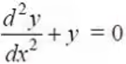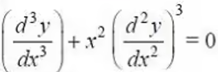The first, second and third equations  involve the highest derivative of first, second and third order respectively. Therefore, the order of these equations are 1, 2 and 3 respectively.
(ii) Degree of a differential equation
To study the degree of a differential equation, the key point is that the differential equation must be a polynomial equation in derivatives, i.e., y′, y″, y″′ etc. Consider the following differential equations: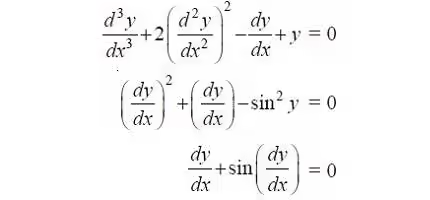We observe that first equation is a polynomial equation in y″′, y″ and y′ with degree 1, second equation  is a polynomial equation in y′ (not a polynomial in y though) with degree of 2. Degree of such differential equations can be defined. But third equation is not a polynomial equation in y′ and degree of such a differential equation can not be defined.
By the degree of a differential equation, when it is a polynomial equation in derivatives, we mean the highest power (positive integral index) of the highest order derivative involved in the given differential equation.
Note: Order and degree (if defined) of a differential equation are always positive integers.
Example Find the order and degree, if defined, of each of the following differential equations:
(i) dy/dx - cosx = 0
(ii) xy d2y/dx2 + x(dy/dx)2 - ydy/dx = 0
(ii) y' + y2 + ey = 0

Solution
(i) The highest order derivative present in the differential equation is dy / dx, so its order is one. It is a polynomial equation in y′ and the highest power raised to  is dy / dx one, so its degree is one.
(ii) The highest order derivative present in the given differential equation is d2y / dx2, so its order is two. It is a polynomial equation in d2y / dx2and dy/dx and the highest power raised to d2y / dx2 is one, so its degree is one.
(iii) The highest order derivative present in the differential equation is y′′′, so its order is three. The given differential equation is not a polynomial equation in its derivatives and so its degree is not defined.
2. Separable Equations
(i) Separable differential equation
y' = A(x)B(y)
Example: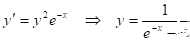RC circuits: Charging: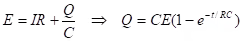Discharging:
IR = Q/C ⇒ Q = Qoe-1/RC
(ii) Linear Differential Equations
y'(x) + p(x)y(x) = q(x)
integrating factor: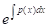Example: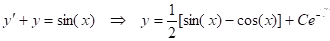y' = 3x2- y/x
y(1) = 5
(iii) Exact Differential Equations
(a) Potential function: For M(x, y) + N(x, y)y' = 0 we can find a ∅(x, y) such that d∅/dx = M and d∅ / dy = N;  is the potential function;  M(x, y) + N(x, y)y' is exact.
(b) Exact differential equation: A potential function exists; general solution: ∅(x, y) =
Example: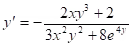Theorem: Test for exactness: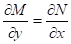dM / dy = dN / dx
Example:  x2 = 3xy + (4xy + 2x)y'= 0
ex sin y - 2x + (ex cosy + 1)y' = 0
(iv) Integrating Factors
(a) Integrating factor: μ(x, y) ‡ 0 such that μM(x,y) + μN(x,y)y' = 0 is exact.
Example: y2 - 6xy + (3xy - 6x2)y' = 0
(b) How to find integrating factor: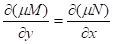Example: x - xy - y'= 0
A. Separable equations and integrating factors: μ= 1/B
B. Linear equations and integrating factors: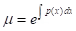(v) Homogeneous and Bernoulli Equations
(a) Homogeneous differential equation: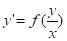; let y = ux separable.
Example: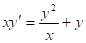.
(b) Bernoulli equation: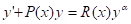y' = P(x)y = R(x)yα; α= 0 linear;α= 1
⇒ separable; otherwise, let v = y1-α ⇒ linear
Example: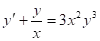.

Higher order linear odes

1. Homogeneous linear odes
An linear ordinary differential equation of order n is said to be homogeneous if it is of the form
an(x)y(n) + an - 1(x)y(n - 1) + .... + a1(x)y' + a0 (x)y = 0
where y'= dy/dx i.e., if all the terms are proportional to a derivative of y (or y itself) and there is no term that contains a function of x alone.
However, there is also another entirely different meaning for a first-order ordinary differential equation. Such an equation is said to be homogeneous if it can be written in the form
dy/dx = F(y/x)
Points to Remember
(i) F(x,y,y', ....y(n)) = 0, a nth order ODE if the nth derivative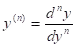of the unknown function y(x) is the highest occurring derivative.
(ii) Linear ODE: y(n) + pn - 1(x)y(n - 1) + ...+ p1(x)y' + p0(x)y = g(x)
(iii) Homogeneous linear ODE: y(n) + pn - 1(x)y(n - 1) + ...+ p1(x)y' + p0(x)y = g(x) = 0
Theorem: Fundamental Theorem for the Homogeneous Linear ODE: For a homogeneous linear ODE, sums and constant multiples of solutions on some open interval I are again solutions on I. (This does not hold for a nonhomogeneous or nonlinear ODE!).
General solution: y= c1y1+...+ cnyn, where y1,....,yn is a basis (or fundamental system) of solutions on I; that is, these solutions are linearly independent on I.
(iv) Linear independence and dependence: n functions y1,....,yn are called linearly independent on some interval I where they are defined if the equation k1y1,....,knyn = 0  on I implies that all k1,....,kn are zero. These functions are called linearly dependent on I if this equation also holds on I for some k1,....,kn not all zero.
Example: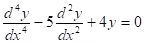.  Sol.:  y = c1e-2x + c2e-x + c3ex + c4e2x
Theorem: Let the homogeneous linear ODE have continuous coefficients p0(x),... , pn-1(x) on an open interval I. Then n solutions y1,....,yn on I are linearly dependent on I if and only if their Wronskian is zero for some x= x0 in I. Furthermore, if W is zero for x= x0, then W is identically zero on I. Hence if there is an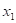in I at which W is not zero, then y1,....,yn are linearly independent on I, so that they form a basis of solutions of the homogeneous linear ODE on I.
Wronskian:(v) Initial value problem: An ODE with n initial conditions y(x0) =K0, y'(x0) = K1, yn-1(x0) = Kn .
2. Homogeneous linear odes with constant coefficients
(i): Substituting y= eλx, we obtain the characteristic equation λn + an-1λn-1 + ...+ a1λ + a0 = 0.
(a) Distinct real roots: The general solution is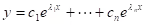Example: y''' - 2y'' - y' + 2y =0.
Solution: y= c1e-x+ c2ex + c3e2x
(b) Simple complex roots: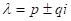, y1 = epx cos(qx), y2 = epx sin(qx) .
Example: y''' - y'' - 100y' + 100y =0.
Solution: y = c1ex + c2 cos10x + c3 sin10x.
(c) Multiple real roots: If λ is a real root of order m, then corresponding linearly independent solutions are: eλx,xeλx, x2eλx,xm-1eλx .
Example: y(5) - 3y(4) + 3y''' - y'' = 0.
Solution: y = c1 + c2x + (c+ c4x + c5x2)ex.
(d) Multiple complex roots: If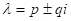are complex double roots, the corresponding linearly independent solutions are:
epx cos(qx), epx sin(qx),xepx cos(qx), xepx sin(qx), .
(ii) Convert the higher-order differential equation to a system of first-order equations.
Example: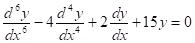.
3. Non - Homogeneous linear odes
(i) y(n) + pn-1(x)y(n-1) + ...+ p1(x)y' + p0(x)y = g(x)  the general solution is of the form: y = yh + yp where yh is the homogeneous solution and yis a particular solution.
(ii) Method of undermined coefficients
Example: y'''+ 3y'' + 3y'+y = 30e
Solution:  y = (c1 + c2x + c3x2)e-x + 5x3e-x
(iii) Method of variation of parameters:
yp = u1y1 +...+ unyn
where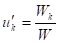, k = 1,...., n.
Example: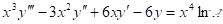.
Solution: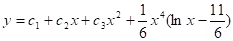.
Offer running on EduRev: Apply code STAYHOME200 to get INR 200 off on our premium plan EduRev Infinity!

54 docs|21 tests

,

,

,

,

,

,

,

,

,

,

,

,

,

,

,

,

,

,

,

,

,

;